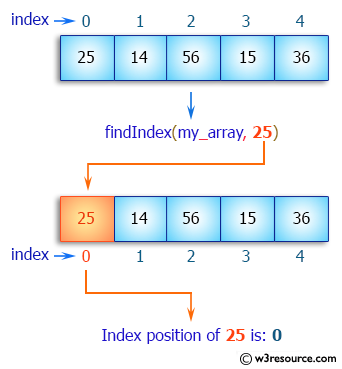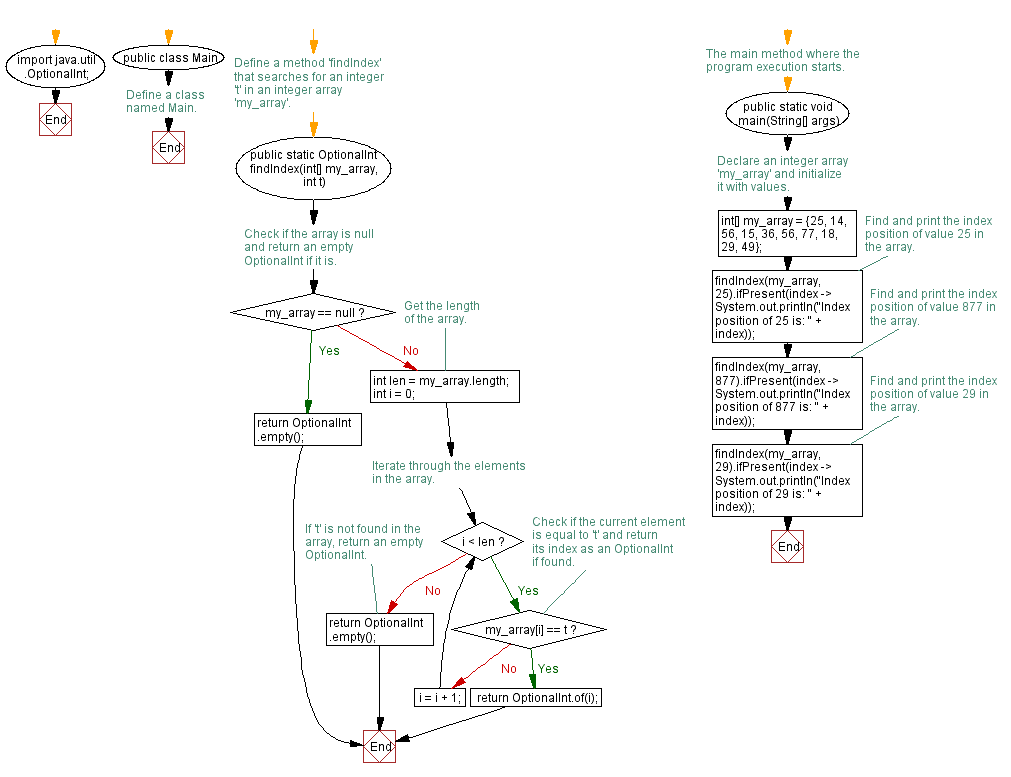﻿ Java exercises: Find the index of an array element - w3resource# Java Array Exercises: Find the index of an array element

## Java Array: Exercise-6 with Solution

Write a Java program to find the index of an array element.

Pictorial Presentation:Sample Solution:

Java Code:

``````public class Exercise6 {
public static int  findIndex (int[] my_array, int t) {
if (my_array == null) return -1;
int len = my_array.length;
int i = 0;
while (i < len) {
if (my_array[i] == t) return i;
else i=i+1;
}
return -1;
}
public static void main(String[] args) {
int[] my_array = {25, 14, 56, 15, 36, 56, 77, 18, 29, 49};
System.out.println("Index position of 25 is: " + findIndex(my_array, 25));
System.out.println("Index position of 77 is: " + findIndex(my_array, 77));
}
}
```
```

Sample Output:

```Index position of 25 is: 0
Index position of 77 is: 6
```

Flowchart:Visualize Java code execution (Python Tutor):

Java Code Editor:

Improve this sample solution and post your code through Disqus

What is the difficulty level of this exercise?

Test your Programming skills with w3resource's quiz.

﻿

## Java: Tips of the Day

countOccurrences

Counts the occurrences of a value in an array.

Use Arrays.stream().filter().count() to count total number of values that equals the specified value.

```public static long countOccurrences(int[] numbers, int value) {
return Arrays.stream(numbers)
.filter(number -> number == value)
.count();
}
```

Ref: https://bit.ly/3kCAgLb